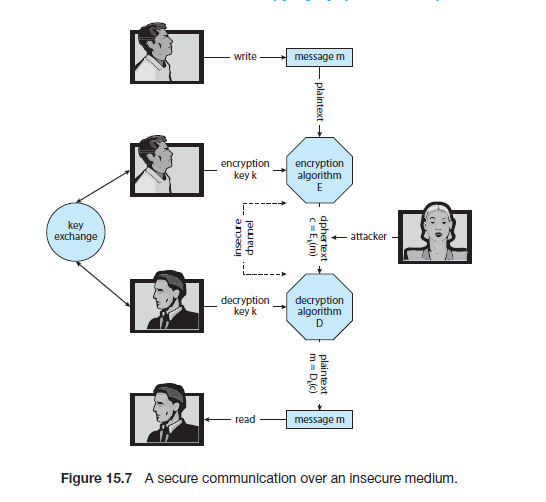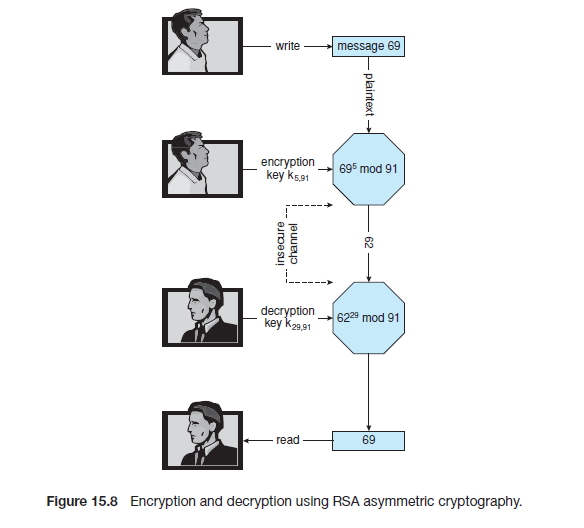# 关于加密，验证, SSL / TLS3.0的基础知识 [OS concepts 搬运工系列]

Posted by Haoxuan (Horace) on 2016-05-28

# Encryption 加密

Because it solves a wide variety of communication security problems, encryption
is used frequently in many aspects of modern computing. It is used to send
messages securely across a network, as well as to protect database data,
files, and even entire disks from having their contents read by unauthorized
entities.

Security, on the other hand, requires not only an adequate protection
system but also consideration of the external environment within which the
system operates.

Breach of confidentiality（保密性）,

Breach of integrity（完整性）,

Breach of availability（可用性，简单的说就是unauthorized destruction未被许可的破坏）,

Theft of service（偷窃服务, 即 unauthorized use of resources私自占有使用资源）,

Denial of service（拒绝服务）.

An encryption algorithm enables the sender of a message to ensure that only a computer possessing a certain key can read the message, or ensure that the writer of data is the only reader of that data.

An encryption algorithm consists of the following components:

• A set K of keys.
• A set M of messages.
• A set C of ciphertexts.
• An encrypting function E : K → (M→C). That is, for each k ∈ K, Ek is a
function for generating ciphertexts from messages. Both E and Ek for any k
should be efficiently computable functions. Generally, Ek is a randomized
mapping from messages to ciphertexts.
• A decrypting function D : K → (C → M). That is, for each k ∈ K, Dk is a
function for generating messages from ciphertexts. Both D and Dk for any
k should be efficiently computable functions.

加密算法由下面部分构成：

• 一个密钥集合K
• 一个消息集合M
• 一个密文集合C
• 一个加密函数 E : K → (M→C). 即，对于每个密钥 k ∈ K, Ek是一个用消息生成密文的函数。对任意k来说， E 和 Ek都是高效的且可计算的函数。大致来说，Ek 是从消息到密文的一个随机映射。
• 一个解密函数 E : K → (C→M). 即，对于每个密钥 k ∈ K, Dk是一个用密文生成消息的函数。对任意k来说， D 和 Dk都是高效的且可计算的函数。大致来说，Dk是从密文到消息的一个随机映射。

An encryption algorithm must provide this essential property: given a
ciphertext c ∈ C, a computer can compute m such that Ek (m) = c only if
it possesses k. Thus, a computer holding k can decrypt ciphertexts to the
plaintexts used to produce them, but a computer not holding k cannot decrypt
ciphertexts. Since ciphertexts are generally exposed (for example, sent on a
network), it is important that it be infeasible to derive k from the ciphertexts.
There are two main types of encryption algorithms: symmetric and
asymmetric.

## Symmetric encryption algorithm and Asymmetric encryption algorithm

### 对称加密：

In a symmetric encryption algorithm, the same key is used to encrypt and to
decrypt. Therefore, the secrecy of k must be protected.data-encryption standard (DES)：64-bit value,a 56-bit key, performing a series of transformations that are based on substitution and permutation operations （基于替换和排列变换）. Work on a block of bits at a time, is known as a block cipher.

triple DES: DES algorithm is repeated three times (two encryptions and one decryption) on the same plaintext using two or three keys—for example, c = Ek3 (Dk2 (Ek1 (m))). When three keys are used, the effective key length is 168 bits (i.e. 56*3).

advanced encryption standard (AES)：another block cipher, use key lengths of 128, 192, or 256 bits and works on 128-bit blocks. 这里block cipher应该是按照固定bit长度加密的意思

RC4: stream cipher based (encrypt and decrypt a stream of bytes or bits rather than a block). This is useful when the length of a communication would make a block cipher
too slow.

### 非对称加密：

In an asymmetric encryption algorithm, there are different encryption and
decryption keys…. Any sender can use that key to encrypt a communication,
but only the key creator can decrypt the communication. This scheme, known
as public-key encryption, was a breakthrough in cryptography.

### Example: RSA Algorithm 举例：RSA 加密算法

In RSA, ke is the public key, and kd is the private key. N is the product of
two large, randomly chosen prime numbers p and q (for example, p and q are
512 bits each). It must be computationally infeasible to derive kd,N from ke,N , so
that ke need not be kept secret and can be widely disseminated. The encryption
algorithm is Eke ,N(m) = mke mod N, where ke satisfies ke kd mod (p−1)(q−1) =1. The decryption algorithm is then Dkd ,N(c) = ckd mod N.

An example using small values is shown in Figure 15.8. In this example, we
make p = 7 and q = 13.We then calculate N = 7∗13 = 91 and (p−1)(q−1) = 72.
We next select ke relatively prime to 72 and < 72, yielding 5. Finally, we calculate
kd such that ke kd mod 72 = 1, yielding 29. We now have our keys: the public
key, ke ,N = 5, 91, and the private key, kd ,N = 29, 91. Encrypting the message 69
with the public key results in the message 62, which is then decoded by theRSA 算法： 数学知识

The use of asymmetric encryption begins with the publication of the public
key of the destination. For bidirectional communication, the source also must
publish its public key. “Publication” can be as simple as handing over an
electronic copy of the key, or it can be more complex. The private key (or “secret
key”) must be zealously guarded, as anyone holding that key can decrypt any
message created by the matching public key.

We should note that the seemingly small difference in key use between
asymmetric and symmetric cryptography is quite large in practice. Asymmetric
cryptography is much more computationally expensive to execute. It is much
faster for a computer to encode and decode ciphertext by using the usual
symmetric algorithms than by using asymmetric algorithms. Why, then, use
an asymmetric algorithm? In truth, these algorithms are not used for general purpose
encryption of large amounts of data. However, they are used not
only for encryption of small amounts of data but also for authentication,
confidentiality, and key distribution, as we show in the following sections.

# Authentication认证

We have seen that encryption offers a way of constraining the set of possible
receivers of a message. Constraining the set of potential senders of a message
is called authentication. Authentication is thus complementary to encryption.

Authentication is also useful for proving that a message has not been modified.

An authentication algorithm using symmetric keys consists of the following components:

• A set K of keys.
• A set M of messages.
• A set A of authenticators.
• A function S : K → (M → A). That is, for each k ∈ K, Sk is a function for
generating authenticators from messages. Both S and Sk for any k should
be efficiently computable functions.
• A function V : K → (M×A→{true, false}). That is, for each k ∈ K, Vk
is a function for verifying authenticators on messages. Both V and Vk for
any k should be efficiently computable functions.

• 一个密钥集合K
• 一个消息集合M
• 一个验证器集合A
• 一个验证器生成函数 S : K → (M→A). 即，对于每个密钥 k ∈ K, Sk 是一个用消息生成验证器的函数。对任意k来说， S和Sk 都是高效的且可计算的函数。
• 一个验证器验证函数 E : K → (M×A→{true, false}). 即，对于每个密钥 k ∈ K, Vk 是一个用来验证特定消息的验证器的函数。对任意k来说， V 和Vk 都是高效的且可计算的函数。

The critical property that an authentication algorithm must possess is this:
for a message m, a computer can generate an authenticator a ∈ A such
that Vk (m, a) = true only if it possesses k. Thus, a computer holding k can generate authenticators on messages so that any computer possessing k can
verify them. However, a computer not holding k cannot generate authenticators
on messages that can be verified using Vk . Since authenticators are generally
exposed (for example, sent on a network with the messages themselves), it
must not be feasible to derive k from the authenticators. Practically, if Vk (m, a)
= true, then we know that m has not been modified, and that the sender of
the message has k. If we share k with only one entity, then we know that the
message originated from k.

Just as there are two types of encryption algorithms, there are two main varieties of authentication algorithms.

### Hash函数

The first step in understanding these algorithms is to explore hash functions. A hash function H(m) creates a small, fixed-sized block of data, known as a message digest or hash value, from a message m. Hash functions work by taking a message, splitting it into blocks, and processing the blocks to produce an n-bit hash. H must be collision resistant —that is, it must be infeasible to find an m = m such that H(m) = H(m ). Now, if H(m) = H(m ), we know that m = m — that is, we know that the message has not been modified. Common message-digest functions include MD5, now considered insecure, which produces a 128-bit hash, and SHA-1, which outputs a 160-bit hash. Message digests are useful for detecting changed messages but are not useful as authenticators. For example, H(m) can be sent along with a
message; but if H is known, then someone could modify m to m and recompute H(m ), and the message modification would not be detected. Therefore, we must authenticate H(m).

### message-authentication code (MAC)消息验证码

• uses symmetric encryption 使用对称加密
• a cryptographic checksum is generated from the message using a secret key 消息的加密校验和通过一个密钥来被生成。
• k is needed to compute both Sk andVk , so anyone able to compute one can compute the other. k用来计算Sk 和Vk ,所以任何能计算一个的人也能计算另外一个。

### digital-signature algorithm数字签名算法

• the authenticators thus produced are called digital signatures 这种算法的验证器也被叫做数字签名。
• Digital signatures are very useful in that they enable anyone to verify the authenticity of the message.数字签名使得任何人可以验证消息的真实性。
• kv is the public key, and ks is the private key. kv 是公钥，ks 是私钥。
• infeasible to derive ks from kv 不可从kv 推导出ks
• Example: RSA digital-signature algorithm ，similar to the RSA encryption algorithm, but the key use is reversed. The digital signature of a message is derived by computing Sks (m) = H(m)ks mod N.The key ks again is a pair <d, N>, where N is the product of two large, randomly chosen prime numbers p and q. The verification algorithm is then
Vkv = ? ( akv mod N = H(m)), where kv satisfies kv ks mod (p − 1)(q − 1) = 1.
• 例子：RSA 数字签名算法, 和RSA加密算法类似，但是key的使用是相反的(译者注：即私钥算出验证器，公钥验证验证器)。一个消息的数字签名Sks (m)是通过计算Sks (m) =H(m)ks mod N 得到的。密钥ks 同样是有序数对<d,N>,N同样是两个巨大的且随机选出的素数p和q之积。验证算法是Vkv = ? ( akv mod N =H(m)),同样kv 满足kv ks mod (p − 1)(q − 1) = 1.

（先写到这里好了，时间有限，回来将后面SSL的部分补上。）